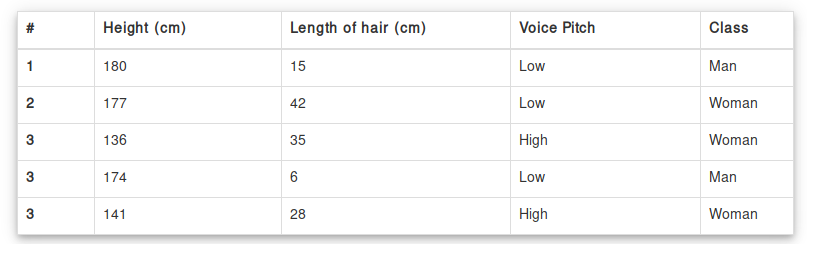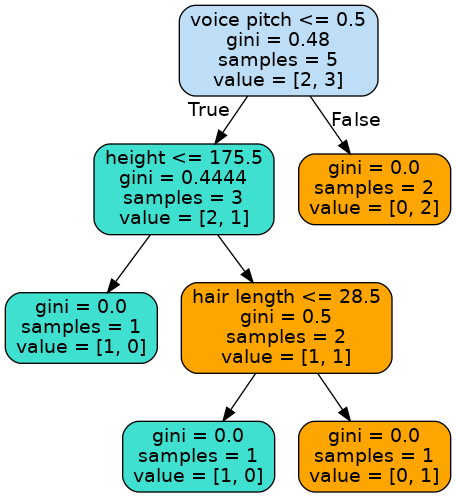tag: decision tree | Python Tutorial

# Tag: decision tree

## Decision tree

A decision tree is one of the many machine learning algorithms. A decision tree is a decision tool. Its similar to a tree-like model in computer science. (root at the top, leaves downwards).

In this article we’ll implement a decision tree using the Machine Learning module scikit-learn. Its one of the many machine learning modules, TensorFlow is another popular one.

Related course:

## Classifiers

Imagine writing a program that has to predict if a picture contains a male or female. You would have to write tons of programming rules. If I’d give you another group of two images, you’d have to create new programming rules all over again. Machine Learning is a better way to solve these problems.

Instead of programatically defining each rule, we use an algorithm that creates the rules for us. This type of algorithm is named a classifier. It takes data as input and shows a label as output.

A practical example of this would be, given an image of a person, the classifier would predict if it’s female or male.

The classifier has these steps:

In this example we’ll use simple arrays as data. In practice you’d often want to have large datasets to make good predictions.

## Decision Tree Visual Example

At every node of the tree, we can turn left or right. Based on numbers we walk the branches. At the end of branches are outcomes. Once the classifier is trained based on this data. We can then use the classifier to make predictions.

A graphical example of a decision tree:Based on numeric input, a computer can decide the output. If its input would be [False, True], it would predict ‘You’.

Related course:

## Install sklearn

If you have not installed sklearn, install it with

also install scipy

## Decision tree

We import tree from sklearn and create the model

Then we create the training data for the classifier / decision tree:

Putting it all together:

## Decision tree visual example

A decision tree can be visualized. A decision tree is one of the many Machine Learning algorithms.
It’s used as classifier: given input data, it is class A or class B? In this lecture we will visualize a decision tree using the Python module pydotplus and the module graphviz

Related course:

If you want to do decision tree analysis, to understand the decision tree algorithm / model or if you just need a decision tree maker - you’ll need to visualize the decision tree.

## Decision Tree

Install
You need to install pydotplus and graphviz. These can be installed with your package manager and pip.
Graphviz is a tool for drawing graphics using dot files. Pydotplus is a module to Graphviz’s Dot language.

Data Collection
We start by defining the code and data collection. Let’s make the decision tree on man or woman. Given input features: “height, hair length and voice pitch” it will predict if its a man or woman.In code that looks like:

Train Classifier
The next step is to train the classifier (decision tree) with the training data.
Training is always necessary for supervised learning algorithms

Decision Tree Visualization
We then visualize the tree using this complete code:

This will save the visualization to the image tree.png, which looks like this:If you want to make predictions, check out the decision tree article.

1